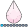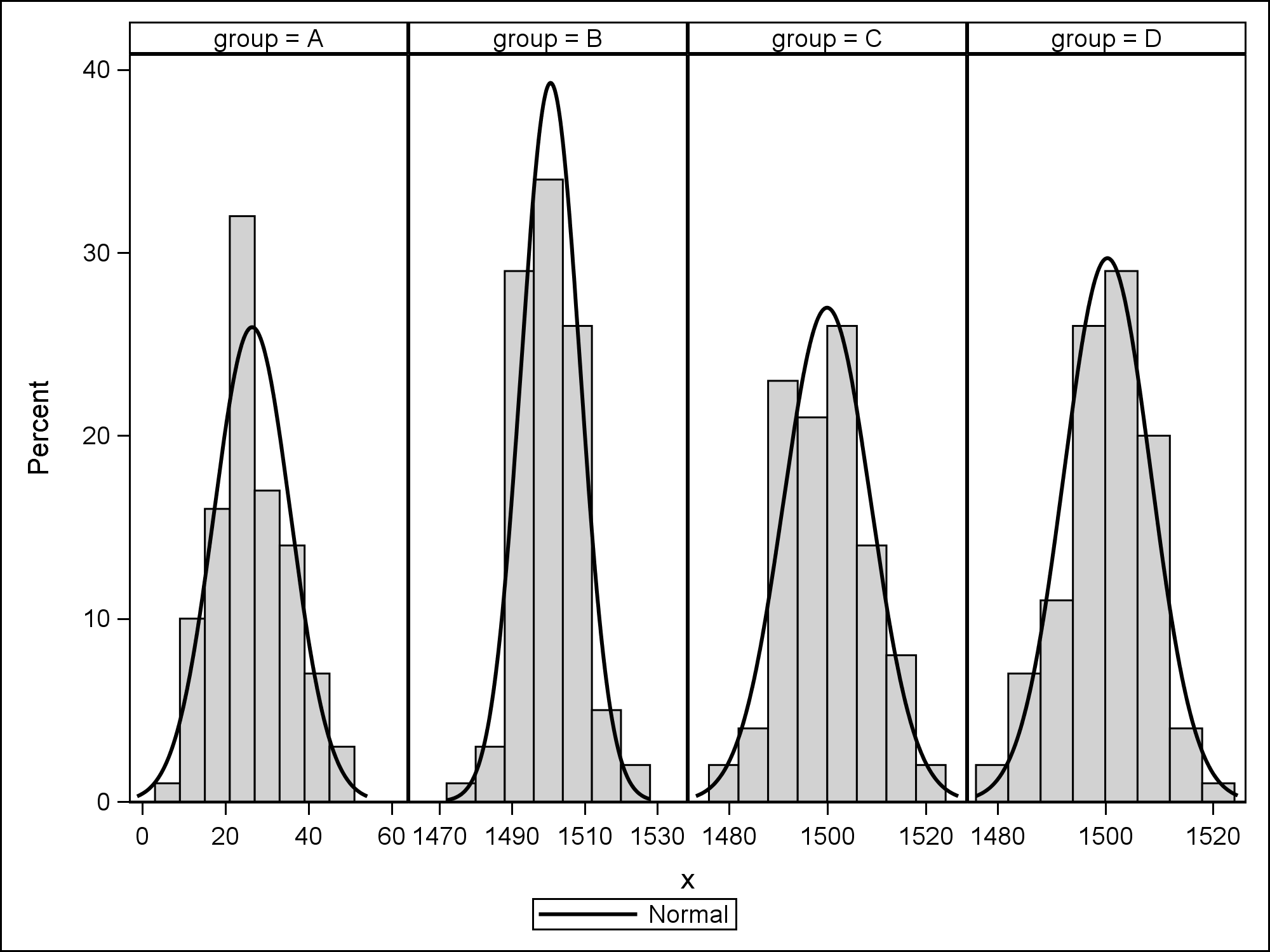## How to create muliple histograms with different x-axis scale?

Hey all. I want to create a table with multiple histograms all of which will have different x-axis scales. Here is some code I have tried on 9.3, where

the same axis scale is automatically picked.

data test;

drop i;

do group='A','B','C','D','E','F','G','H';

do i=1 to 100;

if group='A' then x=25 + 8*rannor(2345);

else x=1500 + 8*rannor(12345);

output;

end;

end;

run;

title 'Comparative plots';

proc univariate data=test noprint;

class group;

var x;

histogram x / vscale=count normal(noprint) ncols=4;

inset normal(mu sigma);

run;

title;

Notice that if I replace 1500 with a number closer to 25, say 30, the plots look much better.

I would appreciate any help

1 ACCEPTED SOLUTION

Accepted Solutionsdjrisks
Barite | Level 11

## Re: How to create muliple histograms with different x-axis scale?

That's fine Stefanos.

You can obtain fully independent axes by using the Lattice Layout in GTL in combination with a Macro.

Please see the code below. Also more work can be done to make the Macro more dynamic, such as calculating the maximum number of groups you have and parsing that to the Macro, and also automatically updating the mvar variables. More work needs to be do also include mu and sigma. Anyway try the code below to obtain independent histograms.

/* Sorting the data */

proc sort data = test;
by group x;
run;

/* Adding a numeric variable to the dataset to identify histogram plot */

data test2;
set test;
by group x;
retain groupn 0;
if first.group then groupn = groupn + 1;
run;

/* Creating Macro Variables for group labels */

proc sql noprint;
select distinct(group) into :lab1 - :lab8
from test2;
quit;

%macro graph(number);

proc template;
define statgraph histogram_with_statistics;
mvar lab1 lab2 lab3 lab4 lab5 lab6 lab7 lab8; /* Including Macro Variables for Labels */
begingraph;
layout lattice /columns = 4 rowdatarange = unionall /* Making y-axis consistent */;

/* Using external axis */
rowaxes;
rowaxis;
rowaxis; /* Will need to add in a rowaxis for each plot */
endrowaxes;

%do i = 1 %to &number;
layout overlay / xaxisopts = (LABEL = LAB&i);
histogram eval(ifn(groupn = &i, x, .));
endlayout;
%end;
endlayout;
endgraph;
end;
run;

%mend;
%graph(8)

ods graphics / reset = all imagename = "Panelled Histogram";

proc sgrender data = test2 template = histogram_with_statistics;
run;

Hope this helps.

Thanks.

8 REPLIES 8djrisks
Barite | Level 11

## Re: How to create muliple histograms with different x-axis scale?

Hello, you can use Proc SGPANEL with the UNISCALE option = to row to help obtain semi indepedent x-axis. Although, you would need to use more code to produce the means and sigma. These statistics can be calculated before hand though, and then placed in your graph. However I believe this is easier using GTL rather than SGPANEL.

Here is SGPANEL code that you can use to produce the (semi independent x-axis) Histograms.

proc sgpanel data = test;

panelby group / columns = 4 uniscale = row;

histogram x;

run;

## Re: How to create muliple histograms with different x-axis scale?

Thanks for the answer djrisks. Unfortunately this doesn't work for me either, since I need fully independent axes. I know that in matlab the subplot command does what I am trying to achieve but I want to produce the plots in sas.djrisks
Barite | Level 11

## Re: How to create muliple histograms with different x-axis scale?

That's fine Stefanos.

You can obtain fully independent axes by using the Lattice Layout in GTL in combination with a Macro.

Please see the code below. Also more work can be done to make the Macro more dynamic, such as calculating the maximum number of groups you have and parsing that to the Macro, and also automatically updating the mvar variables. More work needs to be do also include mu and sigma. Anyway try the code below to obtain independent histograms.

/* Sorting the data */

proc sort data = test;
by group x;
run;

/* Adding a numeric variable to the dataset to identify histogram plot */

data test2;
set test;
by group x;
retain groupn 0;
if first.group then groupn = groupn + 1;
run;

/* Creating Macro Variables for group labels */

proc sql noprint;
select distinct(group) into :lab1 - :lab8
from test2;
quit;

%macro graph(number);

proc template;
define statgraph histogram_with_statistics;
mvar lab1 lab2 lab3 lab4 lab5 lab6 lab7 lab8; /* Including Macro Variables for Labels */
begingraph;
layout lattice /columns = 4 rowdatarange = unionall /* Making y-axis consistent */;

/* Using external axis */
rowaxes;
rowaxis;
rowaxis; /* Will need to add in a rowaxis for each plot */
endrowaxes;

%do i = 1 %to &number;
layout overlay / xaxisopts = (LABEL = LAB&i);
histogram eval(ifn(groupn = &i, x, .));
endlayout;
%end;
endlayout;
endgraph;
end;
run;

%mend;
%graph(8)

ods graphics / reset = all imagename = "Panelled Histogram";

proc sgrender data = test2 template = histogram_with_statistics;
run;

Hope this helps.

Thanks.

## Re: How to create muliple histograms with different x-axis scale?

Switch from CLASS statement to BY statement? Or do you want the graphs paneled?Jay54
Meteorite | Level 14

## Re: How to create muliple histograms with different x-axis scale?

You can use PROC SGPANEL with UNIFORM=ROW.  if you want separate rows of 4 columns, set COLUMNS=4 ROWS=1.

Your output will have multiple panels like this one.

You can use NOVARNAME on the PANELBY statement to remove the "group=" from the headers.

proc sgpanel data=test;

panelby group / uniscale=row columns=4 rows=1 novarname;

histogram x;

density x;

run;## Re: How to create muliple histograms with different x-axis scale?

Thanks djrisks. That is exactly what I was looking for. I believe, however, that there needs to be an easier way to achieve this.

Sanjay with 8 groups, that is 2 rows and 4 columns not all axis will be free.djrisks
Barite | Level 11

## Re: How to create muliple histograms with different x-axis scale?

That's fine stefanos. Great to know that it helps! Unless there is another way that Sanjay know's about I don't believe there is currently a much easier way to achieve this. The reason being that I believe that only SGSCATTER and the LATTICE Layout can produce completely independent axis in each cell.

This could have also been achieved without using Macro's in GTL, i.e. writing out LAYOUT Overlay manually for each of the Histograms you wanted to display, but I thought I'll do it this way incase you wanted to produce more or less Histograms.

Sanjay, if there is currently not a much easier way to produce independent axis for examples similar to above, could you consider adding in future versions of SAS the ability to use independent axis in PROC SGPANEL (or the ability to plot Histograms in SGSCATTER) please?

Thanks.Jay54
Meteorite | Level 14

## Re: How to create muliple histograms with different x-axis scale?

Easier than 4-5 lines of code?  Did you run the code?  for 8 group values, it will create two separate panels of 1x4 histograms.

Discussion stats
• 8 replies
• 4417 views
• 8 likes
• 4 in conversation